Courses

# NCERT Solutions(Part- 5)- Mensuration Class 8 Notes | EduRev

## Class 8 Mathematics by VP Classes

Created by: Full Circle

## Class 8 : NCERT Solutions(Part- 5)- Mensuration Class 8 Notes | EduRev

The document NCERT Solutions(Part- 5)- Mensuration Class 8 Notes | EduRev is a part of the Class 8 Course Class 8 Mathematics by VP Classes.
All you need of Class 8 at this link: Class 8

Exercise 11.3

Question 1. There are two cuboidal boxes as shown in the adjoining figure. Which box requires the lesser amount of material to make?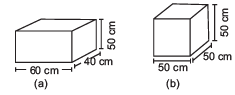Solution: Total surface area of the cuboid (a) = 2[lb + bh + hl]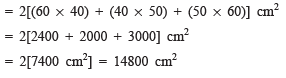Total surface area of the cuboid (b)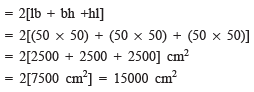Since the total surface area of the second (b) is more.

∴ Cuboid (a) will require lesser material.

Question 2. A suitcase with measures 80 cm *48 cm * 24 cm is to be covered with a tarpaulin cloth. How many metres of tarpaulin of width 96 cm is required to cover 100 such suitcases?

Solution: Total surface area of a suitcase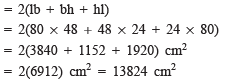∴ Total S.A. of 100 suitcases = 100* 13824 cm2 = 1382400 cm2

Surface area of 1 m of tarpaulin = 100 cm * 96 cm = 9600 cm2

i.e. 1 m of tarpaulin can cover 9600 cm2 surface

∴ 1382400/9600 metres of tarpaulin will cover the total surfaces of 100 suitcases

i.e. 1382400/9600  or 144 metres of tarpaulin will be required to cover 100 suitcases.

Question 3. Find the side of a cube whose surface area is 600 cm2.

Solution: Let the side be x cm.

∴ Total S.A. of the cube = 6 * x2 cm2

But the S.A. of the cube = 600 cm2

∴ 6x2 = 600

or x2 = 600/6 = 100

or x= 102⇒x = 10

∴ The required side of the cube = 10 cm

Question 4. Rukhsar painted the outside of the cabinet of measure 1 m * 2 m * 1.5 m. How much surface area did she cover if she painted all except bottom of the cabinet?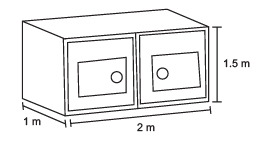Solution: Total surface area = 2[lb * bh + hl]

But bottom is not painted.

i.e. Area not painted = lb

∴ Total S.A. to be painted = 2(bh + hl) + bh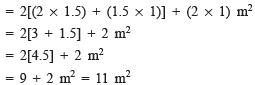Question 5. Daniel is painting the walls and ceiling of a cuboidal hall with length breadth and height of 15 m, 10 m and 7 m respectively. From each can of paint 100 m2 of area is painted. How many cans of paint will she need to paint the room?

Solution: Area to be painted = [Area of 4-walls] + Area of ceiling

= 2[Perimeter * Height] + l * b

= 2[(15 + 10) * 7] + 15 * 10 m2

= 2[25 * 7] + 150 m2

= 2 * 175 + 150 m2

350 + 150 m2 = 500 m2

Since 1 can of paint covers 100 m2 area.

∴ Number of cans required = 500/100 = 5

Question 6. Describe how the two figures at the right are alike and how they are different. Which box has larger surface area?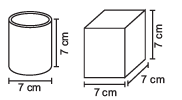Solution: Similarity: Both have the same height.

Difference: Cylinder has curved and circular surfaces.

Cube: All faces are identical squares.

Lateral surface area of the cylinder = 2πrh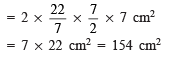Lateral surface area of the cube = 4l2

= 4 * (7 * 7) cm2

= 4 * 49 cm2 = 196 cm2

Obviously the cubical box has larger surface area.

Question 7. A closed cylindrical tank of radius 7 m and height 3 m is made from a sheet of metal. How much sheet of metal is required?

Solution: Here, r = 7 m and h = 3 m

∴ Total surface area = 2πr(r + h)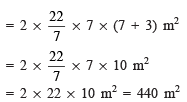Thus, 440 m2 metal is required.

Question 8. The lateral surface area of a hollow cylinder is 4224 cm2. It is cut along its height and formed a rectangular sheet of width 33 cm. Find the perimeter of rectangular sheet.

Solution: ∵ Lateral surface area of a cylinder = 4224 cm2

and let length of the rectangular sheet = l cm

33 * l = 4224

l = 4224/33  = 128 cm

Now perimeter of the rectangular sheet = 2 [Length + Breadth]

= 2 [128 cm + 33 cm]

= 2 *161 cm = 322 cm

Question 9. A road roller takes 750 complete revolutions to move once over to level a road. Find the area of the road if the diameter of a road roller is 84 cm and length is 1 m.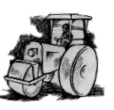Solution: The road roller is a cylinder.

Radius = 84/2  cm = 42 cm

Length (h) = 1 m = 100 cm

∴ Lateral surface area = 2πrh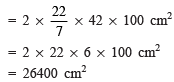∴ Area levelled by the roller in 1 revolution = 26400 cm2

or Area levelled in 750 revolutions = 750 * 26400 cm2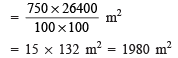Thus, the required area of the road = 1980 m2

Question 10. A company packages its milk powder in cylindrical container whose base has a diameter of 14 cm and height 20 cm. Company places a label around the surface of the container as shown in the figure. If the label is placed 2 cm from top and bottom, what is the area of the label?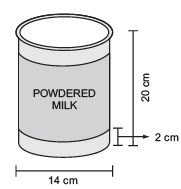Solution: The label is in the shape of a cylinder having

Radius = 14/2 cm = 7 cm and Height = (2 + 2) cm less than the height of the container

or Height of the label = 20 cm – [2 + 2] cm = 16 cm          [∵ The label is placed 2 cm from top and bottom.]

∴ Area of the label = 2πrh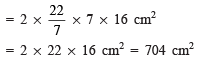93 docs|16 tests

,

,

,

,

,

,

,

,

,

,

,

,

,

,

,

,

,

,

,

,

,

;